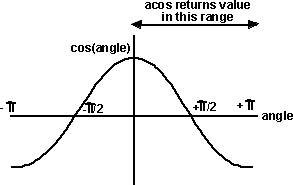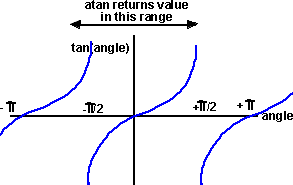# Maths - Trigonometry - Inverse trig functions

The inverse of the sine function is known as arc sine, most math libraries shorten this to asinThe inverse of the cosine function is known as arc cosine, most math libraries shorten this to acosThe inverse of the tangent function is known as arc tangent, most math libraries shorten this to atan or atan2The trig functions are many to one, therefore the inverse trig functions have many possible results. We usually assume that:
acos returns the angle between 0 and pi
asin returns the angle between -pi/2 and pi/2
atan returns the angle between -pi/2 and pi/2
atan2 returns the angle between -pi and pi

## Which value to use?

As we can see from the above graphs, trig functions are many to one. That is, for a given value, it could be produced by many angles. In fact since the graph repeats every 2 pi (360 degrees) there are an infinite number of angles. The values returned by the inverse trig functions are shown above. Usually we want the smallest angle that will represent the required rotation.

## Rectangular To Polar using atan function

For information about polar coordinates see here.

If we want to convert the rectangular coordinates x,y to the polar coordinates θ,r then we can do so as follows:We can calculate r from:

r2 = x2 + y2

and θ from:

tan(θ) = y / x

which gives:

θ = atan(y / x)

## Rectangular To Polar using atan2 function

There are some potential problems with the above approach:

• It does not work for a full range of angles from 0° to 360°, only angles between -90° and +90° will be returned, other angles will be 180° out of phase. For example: the point x=-1,y=-1 will produce the same angle as x=1,y=1 but the above diagram shows that these are in different quadrants.
• points on the vertical axis have x=0, so when we calculate the intermediate result y/x we will get infinity which will generate an exception when calculated on the computer.

Most maths libraries have a atan2(y,x) function which takes both x and y as operands, which allows it to get round the above problems.

In the first quadrant atan2(y,x) is equivalent to atan(y/x) since:

tan(a) = sin(a)/cos(a) = opposite/adjacent = y/x
and

Most maths libraries, for example java, define the order of operands as: atan2(y,x) but some, for example Excel spreadsheet reverse the order of the operands as follows:

ATAN2(x_num,y_num)

X_num is the x-coordinate of the point.

Y_num is the y-coordinate of the point.

Therefore you should always check the order of the order of operands for the maths library you are using.

## Test Program

The following program shows the difference between atan and atan2 results:

```package angles;import java.lang.Math;public class Application1 { public static void value(float x,float y) {
try {
System.out.println("x="+x+" y="+y+" atan(y/x)="+Math.atan(y/x)+"    atan2(y,x)="+Math.atan2(y,x));
} catch (Exception e) {
System.out.println("x="+x+" y="+y+" atan(y/x)=error"+"    atan2(y,x)="+Math.atan2(y,x));
}
} //Main method
public static void main(String[] args) {
value(1,0);
value(1,1);
value(0,1);
value(-1,1);
value(-1,0);
value(-1,-1);
value(0,-1);
value(1,-1);
}
}```

results:

x=1.0 y=0.0 atan(y/x)=0.0 atan2(y,x)=0.0
x=1.0 y=1.0 atan(y/x)=0.7853981633974483 atan2(y,x)=0.7853981633974483
x=0.0 y=1.0 atan(y/x)=1.5707963267948966 atan2(y,x)=1.5707963267948966
x=-1.0 y=1.0 atan(y/x)=-0.7853981633974483 atan2(y,x)=2.356194490192345
x=-1.0 y=0.0 atan(y/x)=-0.0 atan2(y,x)=3.141592653589793
x=-1.0 y=-1.0 atan(y/x)=0.7853981633974483 atan2(y,x)=-2.356194490192345
x=0.0 y=-1.0 atan(y/x)=-1.5707963267948966 atan2(y,x)=-1.5707963267948966
x=1.0 y=-1.0 atan(y/x)=-0.7853981633974483 atan2(y,x)=-0.7853981633974483

 metadata block see also: Correspondence about this page Book Shop - Further reading. Where I can, I have put links to Amazon for books that are relevant to the subject, click on the appropriate country flag to get more details of the book or to buy it from them.Mathematics for 3D game Programming - Includes introduction to Vectors, Matrices, Transforms and Trigonometry. (But no euler angles or quaternions). Also includes ray tracing and some linear & rotational physics also collision detection (but not collision response). Other Math Books

This site may have errors. Don't use for critical systems.